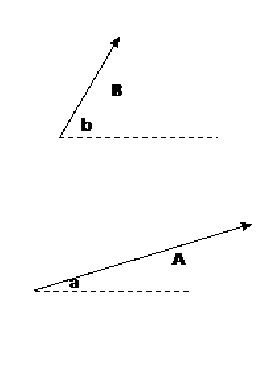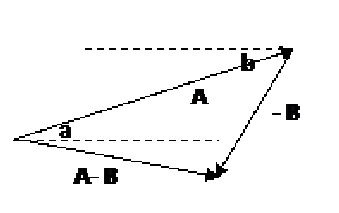Custom Search
If you want to discuss more on this or other issues related to Physics, feel free to leave a message on my Facebook page.

## Tuesday, November 24, 2009

### How to subtract one vector from another?

As we have seen in a previous post a vector is on object that has a magnitude and a direction. We have also seen how to add two vectors together. Today we are going to see how to subtract one vector from another

Let us say that a vector A is as shown and has a magnitude of 4.Then vector  -A will be a vector of the same magnitude and but in the opposite direction like in the diagram belowNow let us have a look at the two vectors A and B of magnitude 4 and 2 respectively.If you want to perform the following subtraction ( A- B ) the first step is to change vector B to vector –B as shown in the diagram below.Now that you have two vectors A and – B you can add them together as you did in the previous post on addition of vectors. You place the tail of vector –B on the tip of vector A  as shown below.The following mathematic is used. A B = A + (-B)  As you can see you add the vector A to the vector –B  to obtain A - B.

The vector AB is a vector that starts from the tail of vector A to the tip of vector –B  as shown in the diagram below.If the magnitude of the two vectors are 4 and 2 respectively then the magnitude of the vector (AB) = 4-2 = 2

If the two vectors were not to be horizontal or vertical then the same method should be used. Let say that we have two vectors as shown below and that you want to determine the vector (AB)

Below are two vectors that are not horizontal or vertical. That is they are not parallel to each other.How would you determine the vector AB ?

First of all you will have to determine the vector –B. As you can see in the diagram below the vector –B has the same magnitude as the vector B but they have opposite direction.Now if you want to determine (AB) you will have to remember that

(AB) = A +(-B)

Which means that if you want to perform the subtraction AB you will have to add the vector A and –B.

Again you will have to add the two vectors by placing the the tail of vector –B on the tip of vector A as shown in the diagram below.As you have seen in the previous examples the vector (AB) is the vector from the tail of A to the tip of –B. As shown in the diagram below.Of course using the angles that –B makes to the horizontal,b, and the angle that A  makes to the horizontal ,a, (AB) can be determined using cosine rule. Also the angle that the vector (AB) makes with the horizontal can be determined.